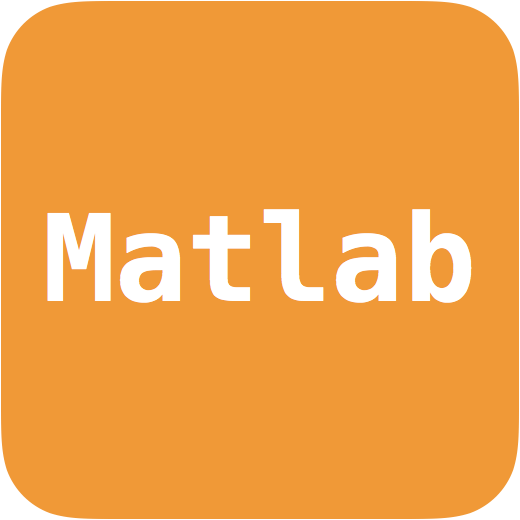# GMCALabAuthors: J. Bobin Language: Matlab and Python Download: Python | Matlab Description: A toolbox for solving Blind Source Separation problems. Notes:

## GMCALab

GMCALab is a set of Matlab toolboxes that focus on solving Blind Source Separation problems from multichannel/multispectral/hyperspectral data. In essence, multichannel data provide different observations of the same physical phenomena (e.g. multiple wavelengths, ), which are modeled as a linear combination of unknown elementary components or sources:

$$\mathbf{Y} = \mathbf{A}\mathbf{S},$$

where $$\mathbf{Y}$$ is the data matrix, $$\mathbf{S}$$ is the source matrix, and $$\mathbf{A}$$ is the mixing matrix. The goal of blind source separation is to retrieve $$\mathbf{A}$$ and $$\mathbf{S}$$ from the knwoledge of the data only.

Generalized Morphological Component Analysis, a.k.a. GMCA, is a BSS method that enforces the sparsity of the sought-after sources:

$$\underset{\mathbf{A},~\mathbf{S}}{\text{argmin}}~\|\mathbf{Y}-\mathbf{A}\mathbf{S}\|_2^2+\|\mathbf{\Lambda}\odot\mathbf{S}\|_1,$$

A lightweight Matlab/Octave version of the GMCALab toolbox is available at this location. Illustrations are provide here.

Please check out the project's GitHub page.

It is worth noting that GMCA provides a very generic framework that has been extended to tackle different matrix factorization problems:

• Non-negative matrix factorization with nGMCA
• Separation of partially correlated sources with AMCA
• The decomposition of hyperspectral data with HypGMCA (available soon)
• The analysis of multichannel data in the presence of outliers with rAMCA at this location (updated the 14/06/16).
• Robust BSS in transformed domains with tr-rGMCA .

We are now developping a python-based toolbox coined pyGMCALab, which is available at this location.